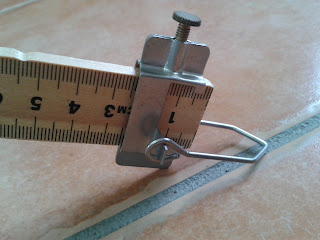## Monday, May 13, 2013

### Rotational Equilibrium is analogous to translational equilibrium, where the sum of the forces are equal to zero. In rotational equilibrium, the sum of the torques is equal to zero. In other words, there is no net torque on the object.#### Another way of expressing the above equation is that torque is the product of the magnitude of the force and the perpendicular distance from the force to the axis of rotation